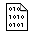## Firefly and PC GAMESS-related discussion club

Learn how to ask questions correctlyDiscontinuous energy curve from XMCQDPT2 results

Panwang Zhou
pwzhou@gmail.com

Dear all,

Recently, I performed a MCSCF(16,14) calculation then following a XMC-QDPT2 calculation on the constructed LIIC curve among the optimized cis-HBDI, CI and trans-HBDI structures, the obtained MCSCF curve is smooth and continuous whereas the obtained XMC-QDPT2 curve is Ādiscontinuous at the CI point (see attachment). I'm not clear why the XMC-QDPT2 curve is discontinuous and how to resolve this problem?

The following is the part of input file:

\$CONTRL SCFTYP=MCSCF RUNTYP=ENERGY EXETYP=RUN MAXIT=50 ICHARG=-1
Ā ĀMULT=1 FSTINT=.T. GENCON=.T. INTTYP=HONDO NOSYM=1
Ā ĀICUT=11 ITOL=30 WIDE=.T. MPLEVL=2 \$END
\$SYSTEM MWORDS=480 TIMLIM=60000.0 KDIAG=0 NOJAC=100 \$END
\$SYSTEM MKLNP=1 NP=12 \$END
\$SMP SMPPAR=.T. HTTNP=1 \$END
\$SCF DIRSCF=.T. FDIFF=.F. NCONV=8 \$END
\$P2P P2P=.T. DLB=.T. \$END
\$TRANS MPTRAN=2 DIRTRF=.T. AOINTS=DIST ALTPAR=.T. MODE=112 \$END
\$MCSCF CISTEP=ALDET FULLNR=.F. SOSCF=.T. MAXIT=100 \$END
\$MCSCF IFORB=.T. \$END
\$DET NCORE=49 NACT=14 NELS=16 NSTATE=6 WSTATE(1)=1,1 DISTCI=12 \$END
\$XMCQDPT NSTATE=2 EDSHFT=0.02 THRGEN=1D-12 MXBASE=90 \$END
\$XMCQDPT HALLOC=.T. \$END
\$XMCQDPT IFORB(1)=-1,1,1 WSTATE(1)=1,1,-0 AVECOE(1)=1,1,-0 \$END
\$BASIS GBASIS=N31 NGAUSS=6 NDFUNC=1 NPFUNC=1 DIFFSP=.TRUE. \$ENDThis message contains the 68 kb attachment [ LIIC_curves.zip ] MCSCF and XMC-QDPT2 CurvesWed May 8 '13 11:50amThis message read 1086 times